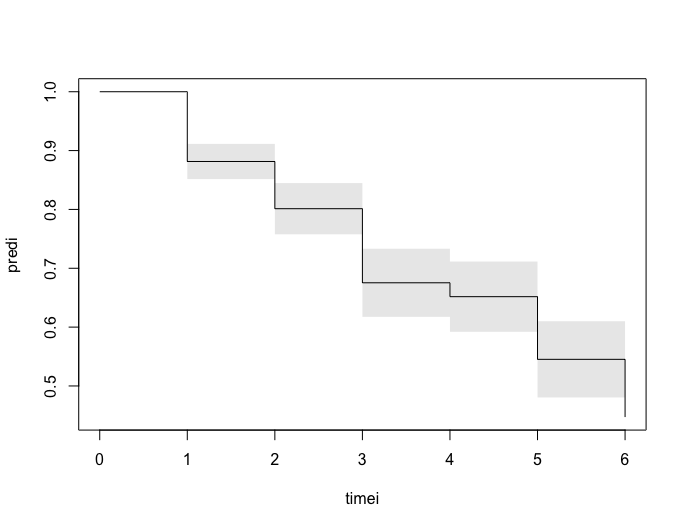# Discrete Inteval Censored survival times

\begin{align*} \mbox{logit}(P(T >t | x)) & = \log(G(t)) + x^T \beta \\ P(T >t | x) & = \frac{1}{1 + G(t) exp( x^T \beta) } \end{align*}

Input are intervals given by $$]t_l,t_r]$$ where t_r can be infinity for right-censored intervals. When truly discrete $$]0,1]$$ will be an observation at 1, and $$]j,j+1]$$ will be an observation at j+1.

Likelihood is maximized: \begin{align*} \prod_i P(T_i >t_{il} | x) - P(T_i> t_{ir}| x). \end{align*}

This model is also called the cumulative odds model \begin{align*} P(T \leq t | x) & = \frac{ G(t) exp( x^T \beta) }{1 + G(t) exp( x^T \beta) }. \end{align*} and $$\beta$$ says something about the OR of probability of being before $$t$$.

The baseline is parametrized as \begin{align*} G(t) & = \sum_{j \leq t} \exp( \alpha_j ) \end{align*}

An important consequence of the model is that for all cut-points $$t$$ we have the same OR parameters for the OR of being early or later than $$t$$.

# Discrete TTP

First we look at some time to pregnancy (discrete survival data) that is right-censored, and set it up to fit the cumulative odds model by constructing the intervals appropriately:

library(mets)

data(ttpd)
dtable(ttpd,~entry+time2)
#>
#>       time2   1   2   3   4   5   6 Inf
#> entry
#> 0           316   0   0   0   0   0   0
#> 1             0 133   0   0   0   0   0
#> 2             0   0 150   0   0   0   0
#> 3             0   0   0  23   0   0   0
#> 4             0   0   0   0  90   0   0
#> 5             0   0   0   0   0  68   0
#> 6             0   0   0   0   0   0 220
out <- interval.logitsurv.discrete(Interval(entry,time2)~X1+X2+X3+X4,ttpd)
summary(out)
#>       Estimate Std.Err     2.5%   97.5%   P-value
#> time1  -2.0064  0.1461 -2.29277 -1.7201 6.466e-43
#> time2  -2.1749  0.1543 -2.47725 -1.8725 3.869e-45
#> time3  -1.4581  0.1496 -1.75132 -1.1648 1.936e-22
#> time4  -2.9260  0.2436 -3.40344 -2.4486 3.078e-33
#> time5  -1.2051  0.1655 -1.52935 -0.8808 3.267e-13
#> time6  -0.9102  0.1790 -1.26103 -0.5594 3.671e-07
#> X1      0.9913  0.1171  0.76175  1.2208 2.557e-17
#> X2      0.6962  0.1156  0.46953  0.9228 1.739e-09
#> X3      0.3466  0.1150  0.12110  0.5721 2.590e-03
#> X4      0.3223  0.1147  0.09749  0.5470 4.952e-03

Now using this discrete survival model we simulate some data from this model

set.seed(1000) # to control output in simulatins for p-values below.
n <- 10000
Z <- matrix(rbinom(n*4,1,0.5),n,4)
outsim <- simlogitSurvd(out$coef,Z) outsim <- transform(outsim,left=time,right=time+1) outsim <- dtransform(outsim,right=Inf,status==0) outss <- interval.logitsurv.discrete(Interval(left,right)~+X1+X2+X3+X4,outsim) summary(outss) #> Estimate Std.Err 2.5% 97.5% P-value #> time1 -2.0151 0.04545 -2.1042 -1.9260 0.000e+00 #> time2 -2.1767 0.04783 -2.2704 -2.0829 0.000e+00 #> time3 -1.4573 0.04628 -1.5480 -1.3666 1.147e-217 #> time4 -2.8678 0.07498 -3.0148 -2.7208 0.000e+00 #> time5 -1.2186 0.05162 -1.3198 -1.1175 3.133e-123 #> time6 -0.8938 0.05581 -1.0031 -0.7844 1.039e-57 #> X1 0.9897 0.03682 0.9176 1.0619 3.444e-159 #> X2 0.6927 0.03641 0.6213 0.7640 1.076e-80 #> X3 0.3211 0.03612 0.2503 0.3919 6.196e-19 #> X4 0.3613 0.03613 0.2905 0.4321 1.537e-23 pred <- predictlogitSurvd(out,se=TRUE) plotSurvd(pred,se=TRUE)And now taking some data and comparing with icenReg. We make the data fully interval censored/discrete by letting also exact obsevations be discrete (thus being in an interval) by modifying the intervals. We consider the interval censored survival times for time from onset of diabetes to to diabetic nephronpathy, and make it discrete by fixing a nuber of fixed cutpoints. test <- 0 if (test==1) { require(icenReg) data(IR_diabetes) IRdia <- IR_diabetes ## removing fully observed data in continuous version, here making it a discrete observation IRdia <- dtransform(IRdia,left=left-1,left==right) dtable(IRdia,~left+right,level=1) ints <- with(IRdia,dInterval(left,right,cuts=c(0,5,10,20,30,40,Inf),show=TRUE) ) } if (test==1) { ints$Ileft <- ints$left ints$Iright <- ints$right IRdia <- cbind(IRdia,data.frame(Ileft=ints$Ileft,Iright=ints$Iright)) dtable(IRdia,~Ileft+Iright) # # Iright 1 2 3 4 5 Inf # Ileft # 0 10 1 34 25 4 0 # 1 0 55 19 17 1 1 # 2 0 0 393 16 4 0 # 3 0 0 0 127 1 0 # 4 0 0 0 0 21 0 # 5 0 0 0 0 0 2 outss <- interval.logitsurv.discrete(Interval(Ileft,Iright)~+gender,IRdia) # Estimate Std.Err 2.5% 97.5% P-value # time1 -3.934 0.3316 -4.5842 -3.28418 1.846e-32 # time2 -2.042 0.1693 -2.3742 -1.71038 1.710e-33 # time3 1.443 0.1481 1.1530 1.73340 1.911e-22 # time4 3.545 0.2629 3.0295 4.06008 1.976e-41 # time5 6.067 0.7757 4.5470 7.58784 5.217e-15 # gendermale -0.385 0.1691 -0.7165 -0.05351 2.283e-02 summary(outss) outss$ploglik
#  -646.1946

fit <- ic_sp(cbind(Ileft, Iright) ~ gender, data = IRdia, model = "po")
#
# Model:  Proportional Odds
# Dependency structure assumed: Independence
# Baseline:  semi-parametric
# Call: ic_sp(formula = cbind(Ileft, Iright) ~ gender, data = IRdia,
#     model = "po")
#
#            Estimate Exp(Est)
# gendermale    0.385     1.47
#
# final llk =  -646.1946
# Iterations =  6
# Bootstrap Samples =  0
# WARNING: only  0  bootstrap samples used for standard errors.
# Suggest using more bootstrap samples for inference
summary(fit)

## sometimes NR-algorithm needs modifications of stepsize to run
## outss <- interval.logitsurv.discrete(Interval(Ileft,Iright)~+gender,IRdia,control=list(trace=TRUE,stepsize=1.0))
}

# SessionInfo

sessionInfo()
#> R version 4.1.1 (2021-08-10)
#> Platform: x86_64-apple-darwin20.4.0 (64-bit)
#> Running under: macOS Big Sur 11.5.2
#>
#> Matrix products: default
#> BLAS:   /usr/local/Cellar/openblas/0.3.17/lib/libopenblasp-r0.3.17.dylib
#> LAPACK: /usr/local/Cellar/r/4.1.1/lib/R/lib/libRlapack.dylib
#>
#> locale:
#>  en_US.UTF-8/en_US.UTF-8/en_US.UTF-8/C/en_US.UTF-8/en_US.UTF-8
#>
#> attached base packages:
#>  stats     graphics  grDevices utils     datasets  methods   base
#>
#> other attached packages:
#>  mets_1.2.9      lava_1.6.11     timereg_2.0.0   survival_3.2-13
#>
#> loaded via a namespace (and not attached):
#>   Rcpp_1.0.7          highr_0.9           bslib_0.2.5.1
#>   compiler_4.1.1      jquerylib_0.1.4     tools_4.1.1
#>   digest_0.6.27       jsonlite_1.7.2      evaluate_0.14
#>  lattice_0.20-44     ucminf_1.1-4        rlang_0.4.11
#>  Matrix_1.3-4        yaml_2.2.1          parallel_4.1.1
#>  mvtnorm_1.1-2       xfun_0.25           fastmap_1.1.0
#>  stringr_1.4.0       knitr_1.33          sass_0.4.0
#>  globals_0.14.0      grid_4.1.1          listenv_0.8.0
#>  R6_2.5.1            future.apply_1.8.1  parallelly_1.27.0
#>  rmarkdown_2.10      magrittr_2.0.1      codetools_0.2-18
#>  htmltools_0.5.2     splines_4.1.1       future_1.22.1
#>  numDeriv_2016.8-1.1 stringi_1.7.4